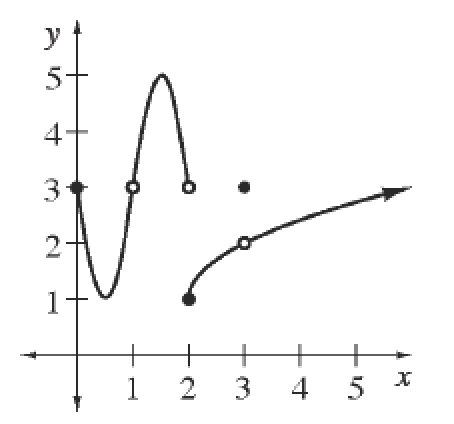### Home > APCALC > Chapter 1 > Lesson 1.2.3 > Problem1-53

1-53.

Convert the following domain and range from interval notation to set notation. Then sketch a possible function with the given domain and range.

• $D=\left(−∞,2\right)\cup\left(2,∞\right)$     $R=\left(−∞,-1\right)\cup\left(-1,∞\right)$

Domain and Range NotationThere are two accepted forms of notation for domain and range. Examine the graph at right. The domain and range of the function shown can be noted in either interval notation or in set notation.

Interval Notation: $D = [0, 1) \cup (1, ∞)$
$R = [1, ∞)$

Set Notation: $D = {x: x ≥ 0 \text{ and } x ≠ 1}$  or  $D = {x: 0 ≤ x < 1 \text{ or } x > 1}$
$R = {y: y ≥ 1}$

The range indicates that this graph never reaches the height of $y = −1$.

The graph of this function could have a hole, jump or vertical asymptote at $y = 2$.